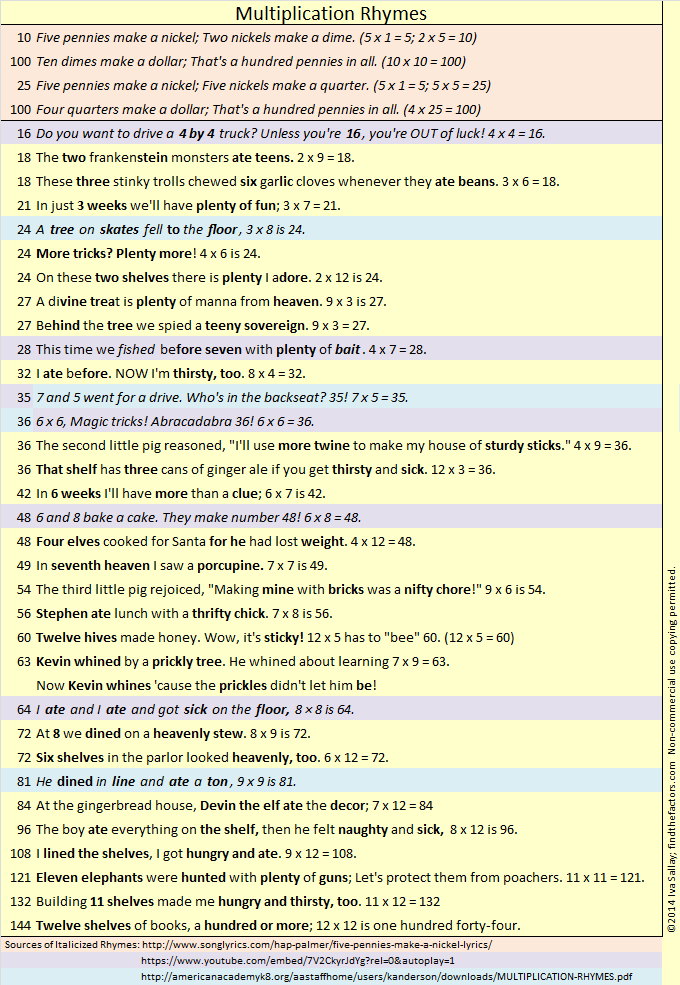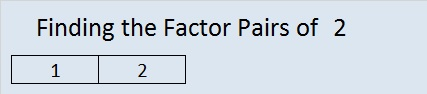# 138 and Divisibility Tricks 4 You

138 is a composite number. Factor pairs: 138 = 1 x 138, 2 x 69, 3 x 46, or 6 x 23. Factors of 138: 1, 2, 3, 6, 23, 46, 69, 138. Prime factorization: 138 = 2 x 3 x 23.138 is never a clue in the FIND THE FACTORS puzzles.

———————————————————————————

After you learned some basic division facts, you probably realized:

• 2 will divide evenly into any EVEN whole number.
• 5 will divide evenly into whole numbers ending in 0 or 5.
• 10 will divide evenly into whole numbers ending in 0.

These three rules are related to each other. All of them are true because we use base ten in our numbering system, and the prime factorization of 10 is 2 x 5.

If you needed to find the factors of a 33-digit whole number, you would be able to tell if 2, 5, or 10 divide evenly into it  just by looking at the last digit. 33-digits is more than a standard calculator can handle, but no matter how many digits a whole number has, as long as you can see the very last one, you can apply those three simple divisibility rules to know if 2, 5, or 10 are factors. Thus you will be able to do something a calculator can’t.

But wait, there are even more divisibility tricks if you can see the last TWO digits of the whole number!• 10 squared, better known as 100, divides evenly into any whole number ending in 00.
• 5 x 5 = 25 which divides evenly into any whole number ending in 00, 25, 50, or 75.
• 2^2 (AKA 4) divides evenly into a whole number if the final two digits can be divided evenly by 4.

How can one tell if the last two digits of a whole number are divisible by 4 (without actually dividing by 4)? I’ll show you how: I’ve put the 25 possible 2-digit multiples of 4 into one of two lists:

• 00, 04, 08, 20, 24, 28, 40, 44, 48, 60, 64, 68, 80, 84, 88
• 12, 16, 32, 36, 52, 56, 72, 76, 92, 96

Notice in the first list ALL the digits are even and the last digit (0, 4, or 8) can be divided evenly by 4.

Then look at the second list. The first digit is always odd and the last digit is either 2 or 6 (the only two even digits that are not divisible by 4).

Hmm. I think we can rewrite the divisibility rule for 4:

• 4 (AKA 2^2) divides evenly into a whole number if the last two digits are even and the final digit is divisible by 4 (the last digit is 0, 4, or 8).
• 4 divides evenly into any whole number whose next to the last digit is odd if the final digit is even but not divisible by 4 (the last digit is 2 or 6).

The rewritten divisibility rule is longer to read but takes a little less time to implement so you will have to decide which version of the rule works best for you. Either trick takes much less time than dividing some really long whole number by 4 or dividing by 2 twice.

Now I’m on to thinking about what the last THREE digits tell us.

# 2 Gross Multiplication FactsThis level TWO multiplication table is only a little more difficult than the table in my previous post.  This puzzle uses 12 factors while the previous one used just 10. To find all these factors you have to be familiar with 144 multiplication facts, a requirement that sounds much more intimidating than it really is. (For example, 3 x 5 and 5 x 3 are counted as 2 different facts.) 144 is a fascinating number.  It is a dozen dozen, otherwise known as a gross. Therefore, completing this puzzle will help you review the gross multiplication facts!   If you haven’t memorized all of the facts, there is a gross way to learn some of the gross multiplication facts.

Edwin A. Anderson Elementary School uses manipulatives and rhymes to teach the multiplication facts.  Their students learn two rhymes a week for sixteen week and are masters of the gross multiplication facts when they are done. Five of the rhymes are actually a little gross:

• 3×3, nine cuts on my knee
• 3×8, 24 horseflies on my plate
• 4×7, 28 spiders are webbin’
• 6×8, fishing bait, count slimy worms all 48
• 7×7, roaches on a vine, yucky and scary all 49

It was a very gross multiplication rhyme about the number 64 that inspired me to collect some favorite rhymes and even write a few rhymes myself. I collected them in one list I titled Multiplication Rhymes:That gross, but inspiring, rhyme about the number 64 and some other gross multiplication rhymes are listed below:

• 18 The two Frankenstein monsters ate teens. 2×9=18
• 18 These three stinky trolls chewed six garlic cloves whenever they ate beans. 3×6=18
• 36 That shelf has three cans of ginger ale if you get thirsty and sick. 12×3=36
• 63 Kevin whined by a prickly tree. He whined about learning 7×9=63. Now Kevin whines ’cause the prickles didn’t let him be!
• 64 I ate and I ate and got sick on the floor, 8×8 is 64.
• 96 The boy ate everything on the shelf. Then he felt naughty and sick. 8×12 is 96.

Knowing all 144 (gross) multiplication facts will help you complete my puzzles.

As you Find the Factors and fill in those gross multiplication facts, there are two things I’d like you to remember:

1. Don’t write anything on the inside of the puzzle until you write down all the factors on the outside of the puzzle.

2. The only numbers allowed in the factor row (top row) and the factor column (1st column) are the numbers from 1 to 12, and all 12 numbers must appear in both places.

Now you can click on 12 Factors 2013-10-31, to find the 6 puzzles appearing in this post.

Now let’s think about the number 2:

2 is the smallest prime number and the only prime that is an even number.

2 is also the largest number that is equal to its number of factors.

In particular situations, there are several prefixes and words that mean two:

• 2 is the second counting number.
• A bicycle is a two-wheeler. A binomial is a polynomial with two terms.
• Two musicians form a duet. Take a look at duet’s synonyms and translations in other languages.
• The playing card with a 2 on it is called the deuce.
• A pair can be two shoes, two eyes, or lots of other possibilities.
• Two people in a relationship are called a couple.

Can you think of any more?

Base 2 uses only the digits 1 and 0 to represent each counting number. Computers use base 2, or binary, as it is also called.

Wikipedia informs us of a Calculus topic about the number 2:

• If you take the sum from 0 to ∞ of b⁻ᵏ, it will converge to b, ONLY when the base number, b, is 2. Thus,
•  ⅟₁ + ⅟₂ + ⅟₄ + ⅟₈ + ⅟₁₆ + ⅟₃₂ + ⅟₆₄ + ⅟₁₂₈ + … = 2

Here is 2’s factoring information:

• 2 is a prime number.
• Prime factorization: 2 is prime.
• The exponent of prime number 2 is 1. Adding 1 to that exponent we get (1 + 1) = 2. Therefore 2 has exactly 2 factors.
• Factors of 2: 1, 2
• Factor pairs: 2 = 1 x 2
• 2 has no square factors that allow its square root to be simplified. √2 ≈ 1.414.How do we know that 2 is a prime number? If 2 were not a prime number, then it would be divisible by at least one prime number less than or equal to √2 ≈ 1.4. Since there are no prime numbers less than or equal to 1.4, we know 2 is a prime number.

When 2 is a clue in the FIND THE FACTOR puzzles, the factors are always 1 and 2.